# 【动态规划入门篇】只需三步解决它

• 2022 年 6 月 02 日
• 本文字数：2638 字

阅读完需：约 9 分钟# 青蛙跳台阶

## 二、题目要求

• 最大运行时间：1s

• 最大运行内存: 128M

### 样例

输入：2  输出：2输入：7  输出：21输入：0  输出：1

### 考察

1.动态规划入门2.建议用时5~15min

## 三、问题分析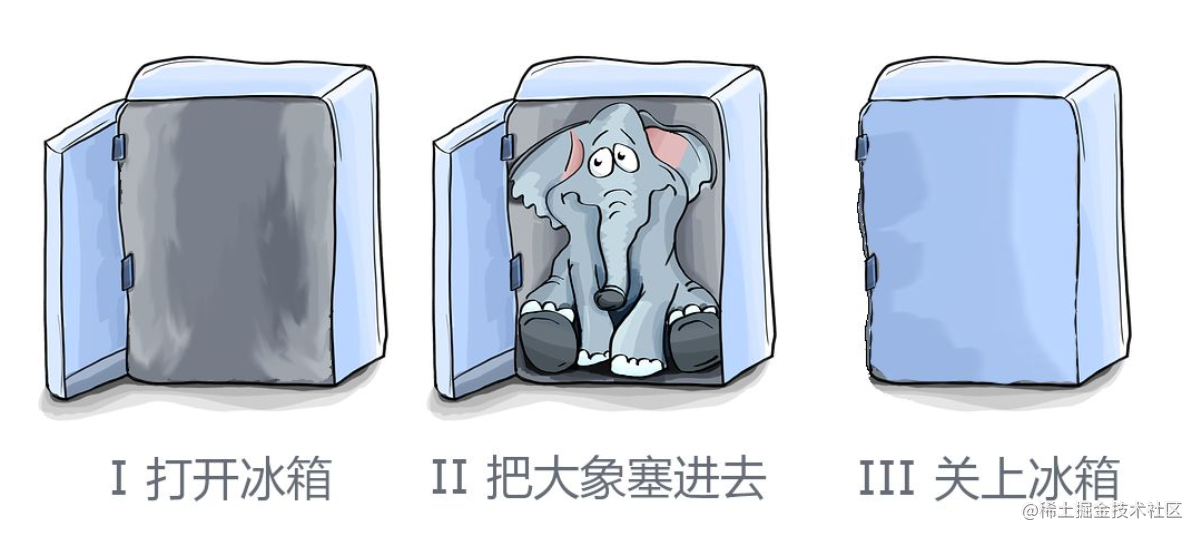### 第二步：变量初始

dp=1             题目样例给了dp=1             1个台阶跨一步就到dp=2             2个台阶可以跨1个台阶再跨1个，也可以一次性跨2个，所以两种方案dp=3             3个台阶每次跨1个、先跨1个再跨2个、先跨2个再跨1个     ......

### 第三步：关系归纳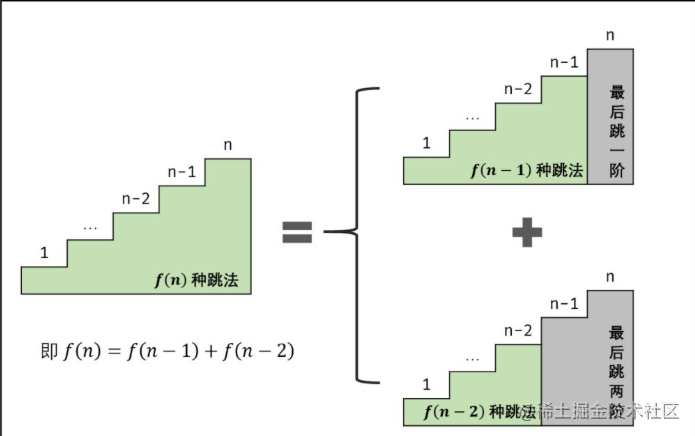dp[n]=dp[n-1]+dp[n-2]。

## 四、编码实现

#include<iostream>using namespace std;int numWays(int n){    if(n<=1) return 1;    int dp[n+1],i;    dp=1,dp=1;//初始化变量    for(i=2;i<=n;i++)    {      //规律        dp[i]=(dp[i-1]+dp[i-2])%1000000007;//取模别忘了    }    return dp[n];//返回结果}int main(){  int k;  cin>>k;//输入台阶数目  cout<<numWays(k);//赋值给动态规划功能的函数  return 0;}

## 五、测试结果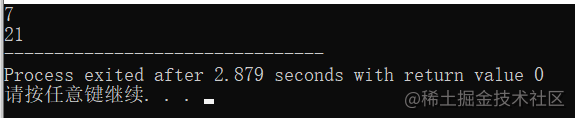# 机器人走迷宫

## 一、问题描述## 二、题目要求

### 考察

1.动态规划中等题型2.建议用时5~15min

### 数据要求

• 1 <= m, n <= 100

• 题目数据保证答案小于等于 2 * 109

### 样例

m=3，n=7     输出28m=3，n=2     输出3m=3，n=3     输出6

## 三、问题分析

### 第二步变量初始：

dp=1 原地踏步不就是吗？一步步写太多了，不想写了，我放一个编译结果图片，偷懒一下。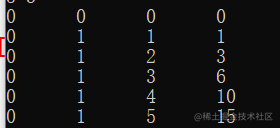## 四、编码实现

#include<iostream>using namespace std;int uniquePaths(int m, int n) {    int i,j,dp={0};//初始化变量    for(i=1;i<=m;i++)//循环判断    {        for(j=1;j<=n;j++)        {            if(i==1&&j==1)                {                    dp=1;//初始化变量                }            else                dp[i][j]=dp[i][j-1]+dp[i-1][j];//规律归纳        }    }//    for(i=0;i<=m;i++)//输出当前数组元素的值//    {//        for(j=0;j<=n;j++)//        {//            cout<<dp[i][j]<<"      ";//        }cout<<"\n";//    }    return dp[m][n];//返回结果}int main(){  int m,n;  cin>>m>>n;//输入数组大小  cout<<uniquePaths(m, n) ;//赋值给动态规划功能的函数  return 0;}

## 五、测试结果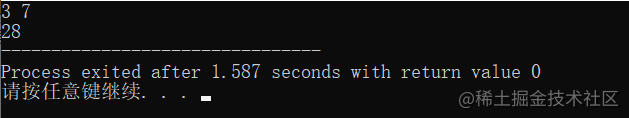# 连续子数组的最大和

## 二、题目要求

• 1 <= arr.length <= 10^5

• -100 <= arr[i] <= 100

• 要求时间复杂度为 O(n)。

输入: nums = [-2,1,-3,4,-1,2,1,-5,4]输出: 6解释: 连续子数组 [4,-1,2,1] 的和最大，为 6。

### 考察

1.动态规划中等题型2.建议用时5~15min

## 三、问题分析

### 第一步含义搞懂：### 第三步规律归纳：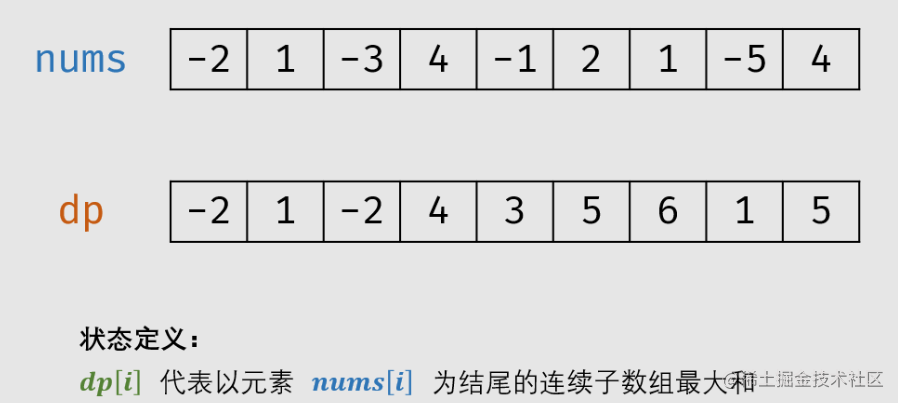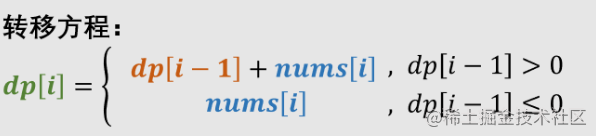## 四、编码实现

#include<iostream>using namespace std;int main(){    long long int i,n,dp,nums,max;//初始化变量    cin>>n;//输入数组大小    for(i=0;i<n;i++)    {      cin>>nums[i];//输入数组数据  }    max=dp=nums;//变量初始    for(i=1;i<n;i++)//循环判断    {        if(dp[i-1]<=0)//负数是本身        {            dp[i]=nums[i];        }        else            dp[i]=nums[i]+dp[i-1];//正数加上上一个        if(dp[i]>max)//是否大于当前max        {            max=dp[i];        }    }    cout<<max;//输出结果  return 0;}

## 五、测试结果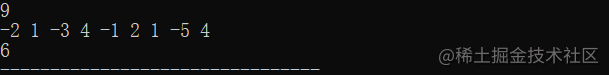## 评论